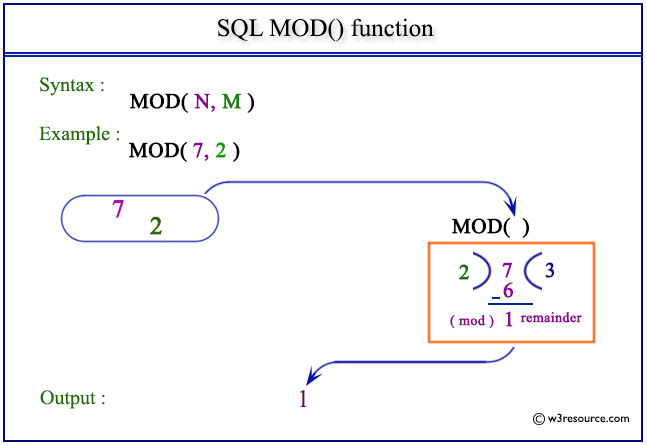# SQL MOD() function

## MOD() function

SQL MOD() function is used to get the remainder from a division. The SQL DISTINCT command along with the SQL MOD() function is used to retrieve only unique records depending on the specified column or expression.

Syntax:

`MOD( dividend, divider )`

PostgreSQL and Oracle

All of above platforms support the SQL syntax of MOD().

MySQL Syntax:

`MOD(dividend,divider); dividend % divider; dividend MOD divider;`

Parameters:

Name Description
dividend A number.
divider A number.

## SQL MOD() function: Pictorial presentationExample:

To get remainder of a division of 25 by 7 from the DUAL table, the following SQL statement can be used :

``````SELECT MOD(25,7)
FROM dual;
``````

Output:

``` MOD(25,7)
----------
4
```
``````SELECT MOD(-25,7)
FROM dual;
``````

Output:

```MOD(-25,7)
----------
-4
```
``````SELECT MOD(25.4,7)
FROM dual;
``````

Output:

```MOD(25.4,7)
-----------
4.4
```
``````SELECT MOD(25.4,7.2)
FROM dual;
``````

Output:

```MOD(25.4,7.2)
-------------
3.8
```
``````SELECT MOD(-25.4,7)
FROM dual;
``````

Output:

```MOD(-25.4,7)
------------
-4.4
```
``````SELECT MOD(25,0)
FROM dual;
``````

Output:

``` MOD(25,0)
----------
25;
```

## SQL MOD() function with distinct

Sample table: customer

To get unique remainder of a division of 'receive_amt' by 'grade' from the 'customer' table, the following SQL statement can be used :

``````SELECT DISTINCT(MOD(receive_amt,grade))
FROM customer;
``````

Output:

```(MOD(RECEIVE_AMT,GRADE))
------------------------
1
2
6000
0
```

## SQL MOD() function with where

Sample table: customer

To get data of 'cust_name', 'opening_amt', 'receive_amt' and remainder of the division of 'opening_amt' by 'receive_amt' from the 'customer' table with following conditions -

1. 'opening_amt' must be more than 'receive_amt',

2. remainder of the division of 'opening_amt' by 'receive_amt' must be more than or equal to 1000,

the following SQL statement can be used :

``````SELECT cust_name,opening_amt,receive_amt,
FROM customer
``````

Output:

```CUST_NAME                                OPENING_AMT RECEIVE_AMT MOD(OPENING_AMT,RECEIVE_AMT)
---------------------------------------- ----------- ----------- ----------------------------
Holmes                                          6000        5000                         1000
Yearannaidu                                     8000        7000                         1000
Shilton                                        10000        7000                         3000
Charles                                         6000        4000                         2000
Martin                                          8000        7000                         1000
Ramesh                                          8000        7000                         1000
```

Note: Outputs of the said SQL statement shown here is taken by using Oracle Database 10g Express Edition.

Here is a slide presentation which covers the SQL arithmetic functions.

Practice SQL Exercises